Integration by specific formulaes - Formula 6

Chapter 7 Class 12 Integrals
Concept wise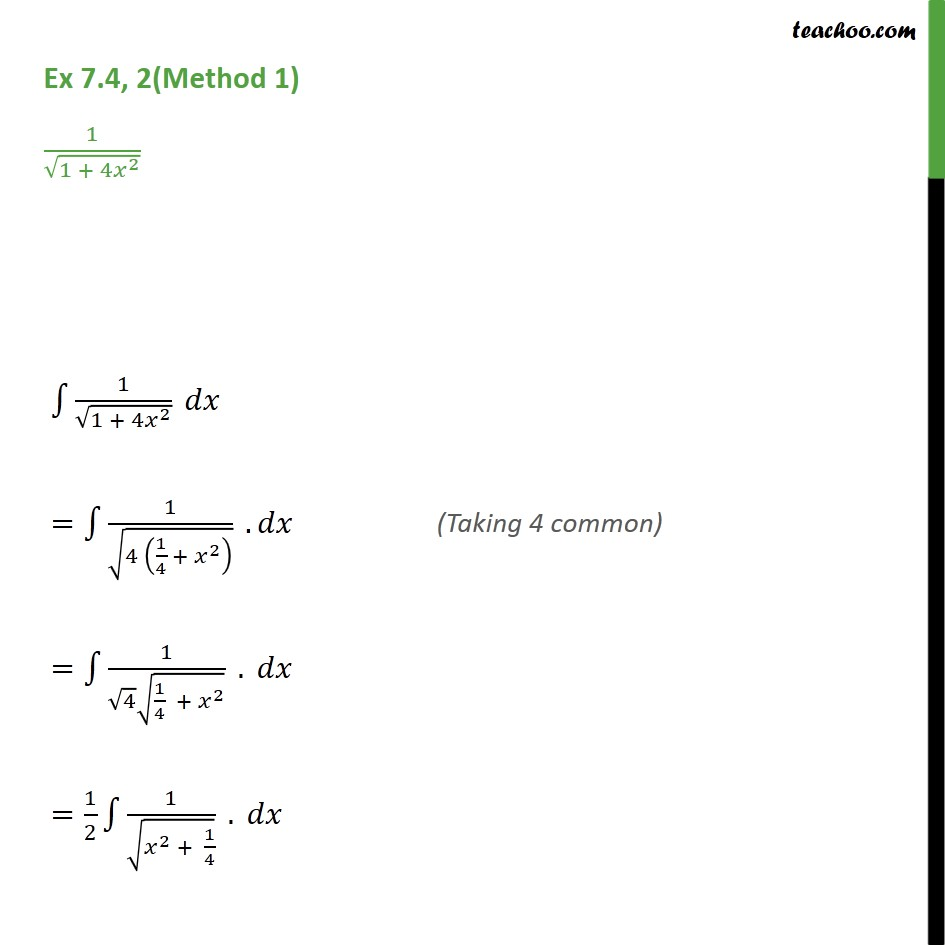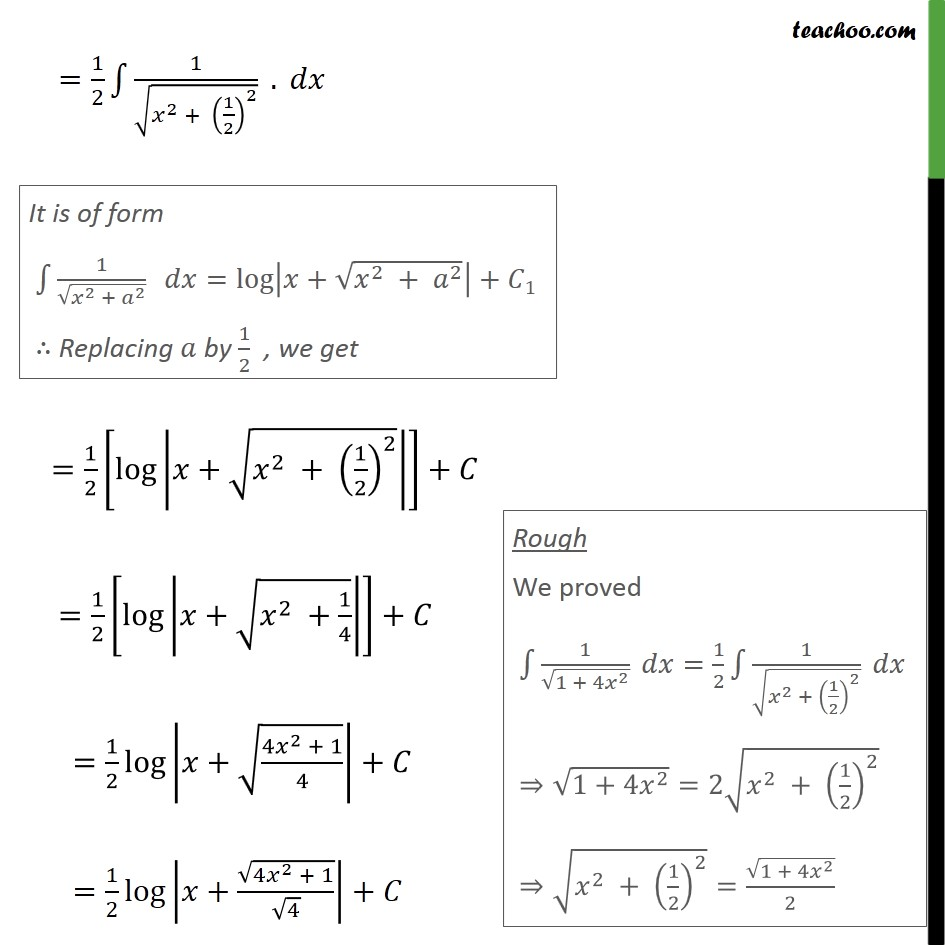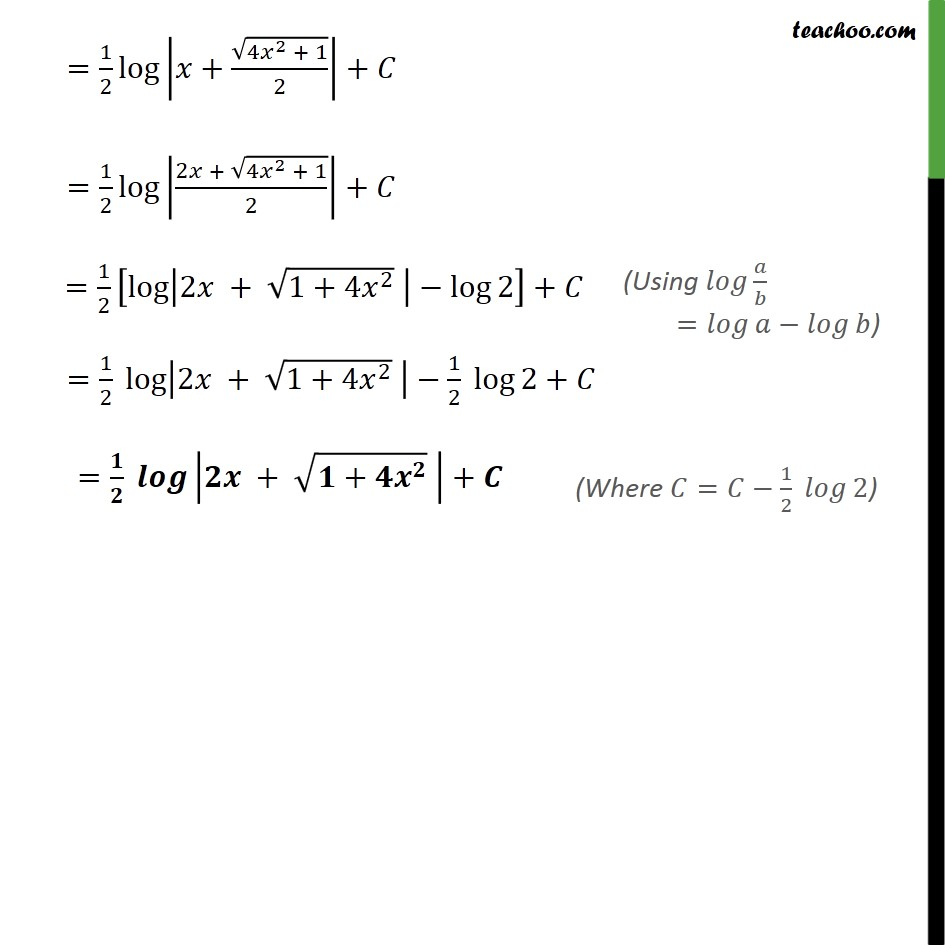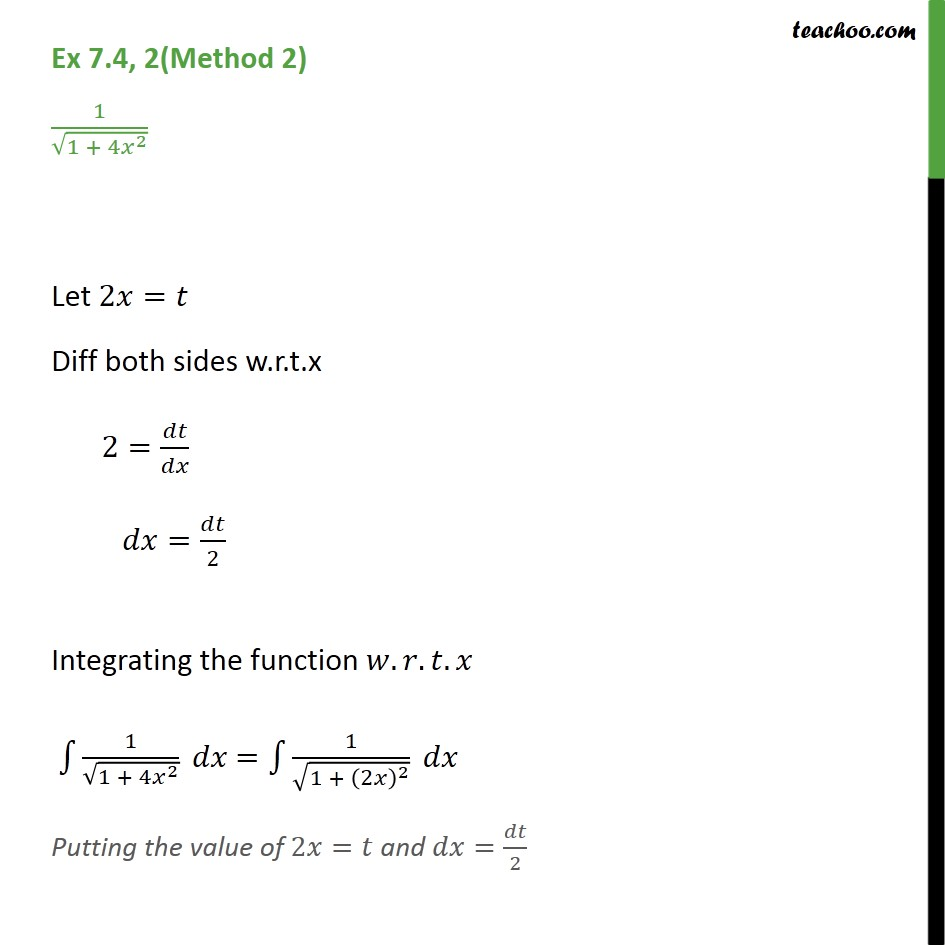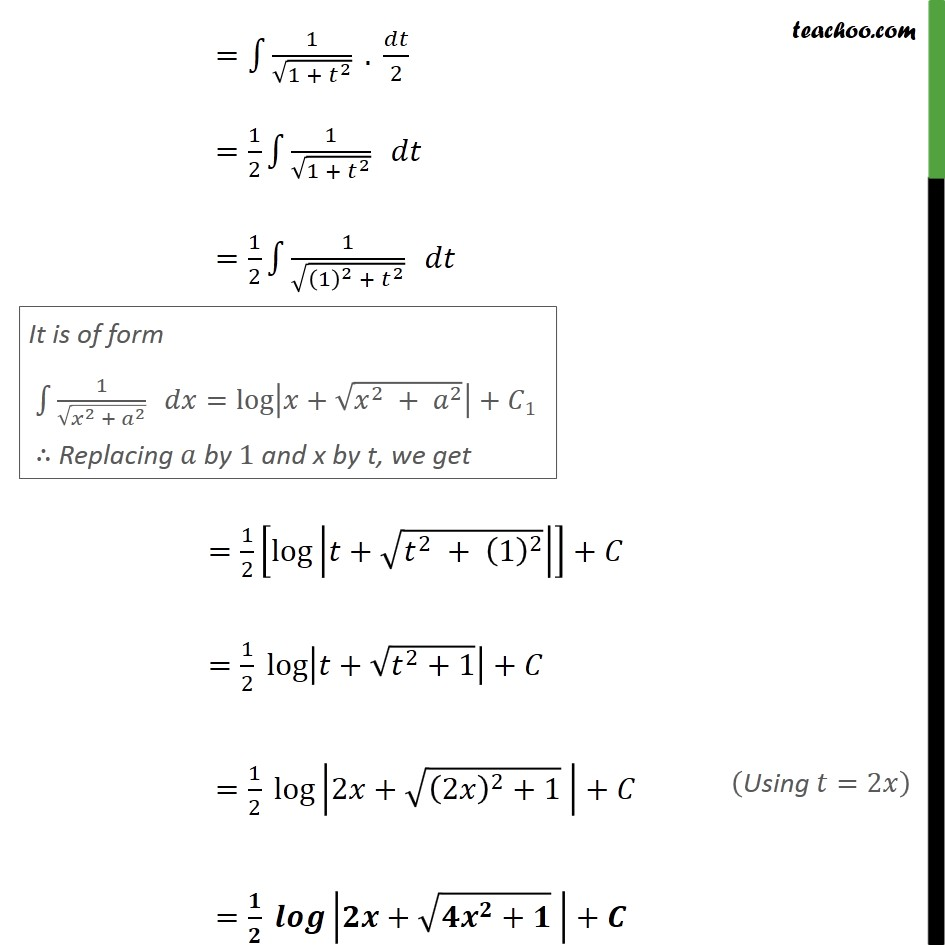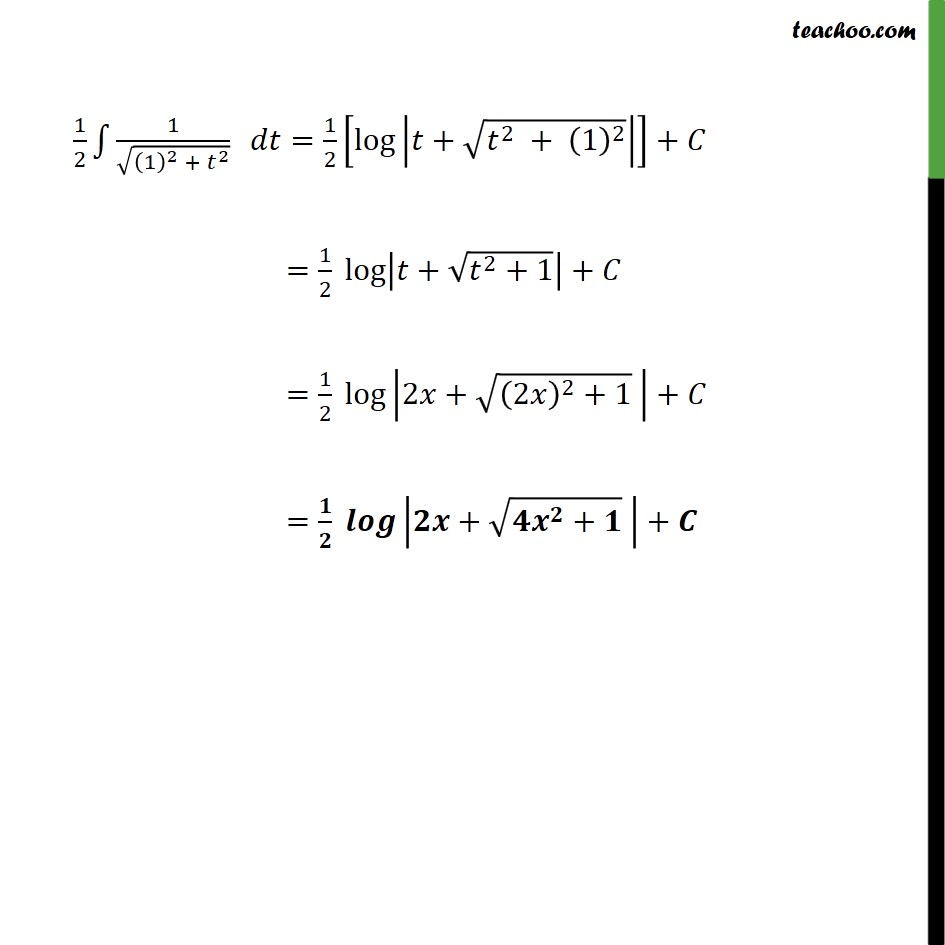Learn in your speed, with individual attention - Teachoo Maths 1-on-1 Class

### Transcript

Ex 7.4, 2(Method 1) 1/√(1 + 4𝑥^2 ) ∫1▒1/√(1 + 4𝑥^2 ) 𝑑𝑥 =∫1▒1/√(4 (1/4 + 𝑥^2 ) ) . 𝑑𝑥 =∫1▒1/(√4 √(1/4 + 𝑥^2 )) . 𝑑𝑥 =1/2 ∫1▒1/√(𝑥^2 + 1/4) . 𝑑𝑥 =1/2 log⁡|𝑥+√(4𝑥^2 + 1)/2|+𝐶 =1/2 log⁡|(2𝑥 + √(4𝑥^2 + 1))/2|+𝐶 =1/2 [log⁡|2𝑥 + √(1+4𝑥^2 ) |−log⁡2 ]+𝐶 =1/2 log⁡|2𝑥 + √(1+4𝑥^2 ) |−1/2 log⁡2+𝐶 =𝟏/𝟐 𝒍𝒐𝒈⁡|𝟐𝒙 + √(𝟏+𝟒𝒙^𝟐 ) |+𝑪